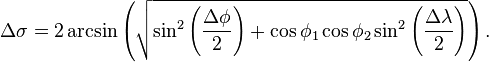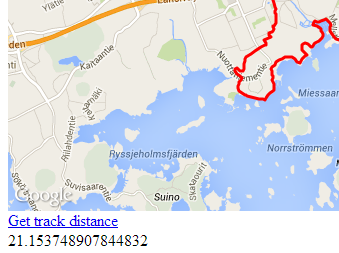# jQuery and GIS distance in MariaDB

I’ve continued building on my MariaDB GIS and node.js example application that I wrote about two weeks back, https://blog.mariadb.org/node-js-mariadb-and-gis/. The application shows how to load GPX information into MariaDB, using some MariaDB GIS functionality, and making use of the node.js platform together with MariaDB’s non-blocking client.

With the GPX data converted into GIS points in the MariaDB database, I wanted to further expand a little on both the GIS aspect and also look at how some additional data could be shown in the application by using jQuery’s Ajax calls to update a piece of the web based application UI.

To start with, an interesting thing to do when you have a bunch of GIS points in a database table is to do distance calculation with the end result being to get the complete distance of the track formed by the points. There of course exists a bunch of different formulas for this, but since MariaDB yet doesn’t have the third coordinate in GIS, which is altitude (or elevation), I chose to use the concept of the Great Circle Distance, https://en.wikipedia.org/wiki/Great-circle_distance  and the Haversine approach. The algorithm for counting the distance between two points in this way is:Since we need to make the distance calculations for all distances between the points it makes sense to create a database function for counting the distance between two points:

```CREATE FUNCTION earth_circle_distance(point1 point, point2 point) RETURNS double
DETERMINISTIC
begin
declare lon1, lon2 double;
declare lat1, lat2 double;
declare td double;
declare d_lat double;
declare d_lon double;
declare a, c, R double;

set lon1 = X(GeomFromText(AsText(point1)));
set lon2 = X(GeomFromText(AsText(point2)));
set lat1 = Y(GeomFromText(AsText(point1)));
set lat2 = Y(GeomFromText(AsText(point2)));

set d_lat = radians(lat2 - lat1);
set d_lon = radians(lon2 - lon1);

set lat1 = radians(lat1);
set lat2 = radians(lat2);

set R = 6372.8; -- in kilometers

set a = sin(d_lat / 2.0) * sin(d_lat / 2.0) + sin(d_lon / 2.0) * sin(d_lon / 2.0) * cos(lat1) * cos(lat2);
set c = 2 * asin(sqrt(a));

return R * c;
end```

With the function in place in the database, we can test it with a query that actually will be the base of the query the application will be using to retrieve the distance for the whole track:

```SELECT
t1.pointId AS point1Id,
t2.pointId AS point2Id,
SUM(earth_circle_distance(t1.gpsPoint, t2.gpsPoint)) AS distance
FROM
trackpoints t1
INNER JOIN
trackpoints t2
ON t2.pointId =(t1.pointId + 1)
WHERE t1.pointId = 8643;```

In the SELECT query I’ve given the id of the first point of the track and then done an INNER JOIN over the same table to be able to get the second point and calculating the distance between the points. I’ve made sure when inserting the points into the database that they are ordered in such a way that the next point on a track after the previous one always has the following pointId, so that I for example now in this case can tell the query that the join is done on pointId + 1. The outcome of running the SELECT –query is:

```+----------+----------+----------------------+
| point1Id | point2Id | distance             |
+----------+----------+----------------------+
|     8643 |     8644 | 0.002970063766987173 |
+----------+----------+----------------------+```

The next step is to do this for every point on the track and sum the distances together to get the full length of the track. This is done by changing the query slightly so that there is no specific pointId restriction and making use of the SUM function to sum all the point distances:

```SELECT SUM(earth_circle_distance(t1.gpsPoint, t2.gpsPoint)) AS distance
FROM trackpoints t1 INNER JOIN trackpoints t2
ON t2.pointId =(t1.pointId + 1)
WHERE t1.trackId = 1;```

With that in place, let’s move to the application side. For the background of setting the whole application up in node.js, please refer to my previous blog post, https://blog.mariadb.org/node-js-mariadb-and-gis/. On the application side I’ll start with defining a new URL mapping through which the distance information will be retrieved. This happens in app.js where a new row is added to the URL mapping section:

`app.get('/trackinfo', common.trackInfo);`

In the URL mapping the common.trackInfo –function is called, which is a really simple function just calling data methods for getting a database connection, querying the distance and closing the database connection. Inside the data method [name of method for retrieving distance] the SELECT query for summing up the distances between the points can be seen. The only parameter being given to the query is trackId, which is read from the URL querystring in the normal node.js way of req.param(“trackId”).

On the UI side, let’s make use of the page that plots the track onto Google Maps. In that web page Google’s implementation of jQuery is used, which can be seen in the source code:

`<script src="http://ajax.googleapis.com/ajax/libs/jquery/1.10.2/jquery.min.js" type="text/javascript"></script>.`

Also notice in the source code how the points that are being plotted onto the map are requested through a \$.getJSON function call. I’m going to do something similar for getting the distance and displaying it. First though let’s create a link through which the retrieval of the distance is fired and add a placeholder for the distance information by adding a div –block in the HTML:

```<a href="javascript:void(0);" onclick="getTrackInfo(1)">Get track distance</a>
<div id="track_info"></div>```

With that in place let’s take a look at the jQuery function that will get us the distance:

```function getTrackInfo(id) {
var url = "/trackinfo?trackId=" + id;

\$.getJSON(url, function (data) {
\$('#track_info').html(data.distance);
});
}```

The function calls the url /trackinfo URL mapping, which returns a JSON formatted output holding the distance information. The distance is easily picked out from JSON and finally placed in the div –block.As you can see, it’s very straight forward to use MariaDB with node.js and make use of jQuery. Also there are many interesting things that can be done by using the GIS capabilities of MariaDB. In MariaDB 10.1 the third coordinate will be present, which then means that the height differences could be considered in the distance calculations and the outcome would be more precise. In this particular case we were looking at a GPX track from a half marathon run I did. I had a running watch on me, which gathered the information for the track. It showed the final distance of the run to be 21.23 kilometers, while the distance counted with the Haversine -algorithm approach was 21.15 kilometers. The difference could very well be that the watch actually included the altitude into the calculations, but I’m of course not sure about that since I don’t know what algorithms it uses.

This example application’s source code is available on Github. Try it out if you’re interested in MariaDB GIS or using node.js and jQuery with MariaDB.

### 1 Comment

1.Patrik Backman
2014-02-07

Very cool!
Btw: you ran passed my house – You should have dropped in for a drink.
What was your finishing time?

### No Pings Yet